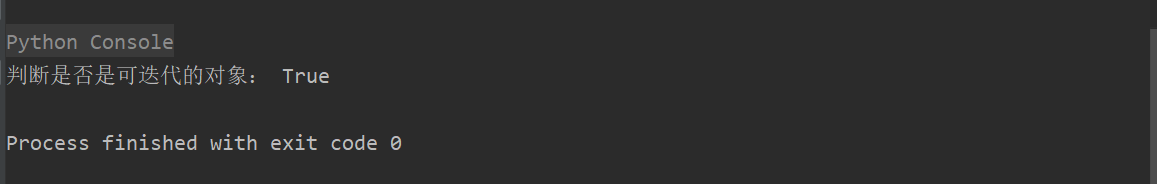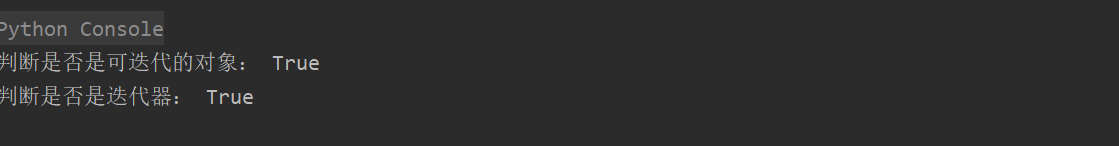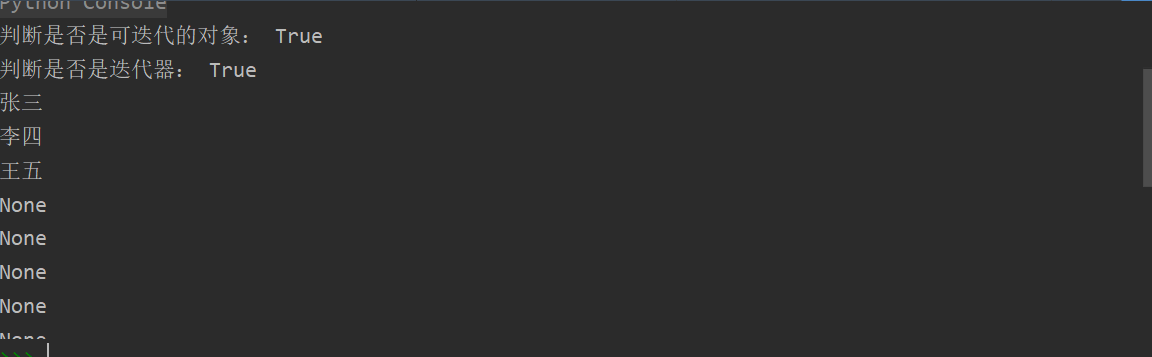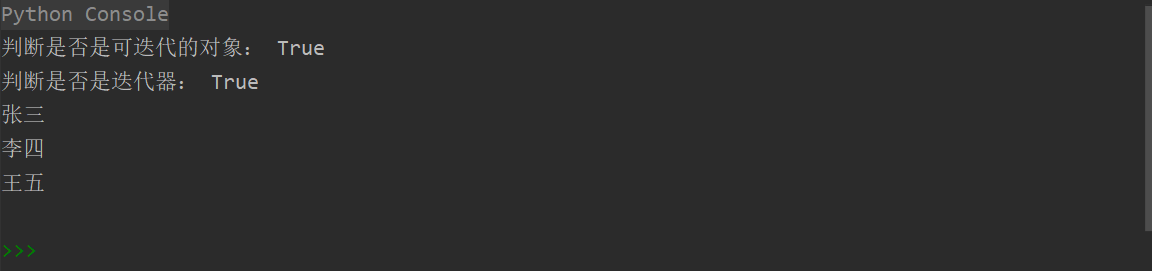### python迭代器详解

``mylist = [1,2,3,4,5]mydirt = {    "name":"张三",    "sex":"男",    "age":18    }    mytuple = (1,2,3,4,5)myset = {1,2,3,3,4} for i in mylist:    print(i) for i in mytuple:    print(i) for i in myset:    print(i) for i,j in mydirt.items():    print("%s:%s" % (i,j))``

``from collections import Iterable  class Classmate(object):    """定义一个同学类"""     def __init__(self):        self.name = list()        self.name_num = 0     def add(self,name):        self.name.append(name)        def __iter__(self):        pass     class1 =  Classmate()class1.add("张三")class1.add("李四")class1.add("王五") print("判断是否是可迭代的对象：", isinstance(class1,Iterable))``1.一个类（对象）只要含有“__iter__”、”__next__”两个方法，就将其称为迭代器。__iter__方法返回一个特殊的迭代器对象，而这个迭代器对象自动实现了_next__方法，并返回一个值，最后通过抛出异常StopIteration来结束迭代。我们来给上一个例子增加__next__方法：

``from collections import Iterablefrom collections import Iterator  class Classmate(object):    """定义一个同学类"""     def __init__(self):        self.name = list()        self.name_num = 0     def add(self,name):        self.name.append(name)        def __iter__(self):        pass     def __next__(self):       pass  class1 =  Classmate()class1.add("张三")class1.add("李四")class1.add("王五") print("判断是否是可迭代的对象：", isinstance(class1,Iterable)) print("判断是否是迭代器：", isinstance(class1,Iterator))``2.只是名义上的 可迭代对象/迭代器 还不够，具有相应的功能才算是完整。首先，对于__iter__方法，它需要具有一个可以返回一个迭代器对象的功能（这个对象可以是自己(前提是本身就是一个迭代器），也可以是其它迭代器）；对于__next__方法，它需要标记并返回下一个迭代器对象。代码如下（为防止迭代速度过快，我们添加sleep来控制速度）：

``from collections import Iterablefrom collections import Iteratorimport time  class Classmate(object):    """定义一个同学类"""     def __init__(self):        self.name = list()        self.name_num = 0     def add(self,name):        self.name.append(name)        def __iter__(self):        return self   # 返回本身     def __next__(self):              # 记忆性返回数据       if self.name_num < len(self.name):           ret = self.name[self.name_num]           self.name_num += 1           return ret   class1 =  Classmate()class1.add("张三")class1.add("李四")class1.add("王五") print("判断是否是可迭代的对象：", isinstance(class1,Iterable)) print("判断是否是迭代器：", isinstance(class1,Iterator)) for name in class1:    print(name)    time.sleep(1)````from collections import Iterablefrom collections import Iteratorimport time  class Classmate(object):    """定义一个同学类"""     def __init__(self):        self.name = list()        self.name_num = 0     def add(self,name):        self.name.append(name)        def __iter__(self):        return self   # 返回本身     def __next__(self):       if self.name_num < len(self.name):           ret = self.name[self.name_num]           self.name_num += 1           return ret         # 抛出异常，当循环后自动结束       else:           raise StopIteration  class1 =  Classmate()class1.add("张三")class1.add("李四")class1.add("王五") print("判断是否是可迭代的对象：", isinstance(class1,Iterable)) print("判断是否是迭代器：", isinstance(class1,Iterator)) for name in class1:    print(name)    time.sleep(1)````a = 0b = 1myFibonacci = list() nums = int(input("请输入需要生成Fibonacci数列项的个数：")) i = 0while i < nums:    myFabonacci.append(a)    a,b = b,a+b    i += 1 for num in myFibonacci:    print(num)``

``class Fibonacci(object):    """斐波那契数列得迭代器"""    def __init__(self,nums):        self.nums = nums   # 传入参数，生成斐波那契数列的个数        self.a = 0           self.b = 1        self.i =0    # 用于记忆生成的个数    def __iter__(self):        return self     def __next__(self):                  ret = self.a   # 记忆第一个数        if self.i < self.nums:            self.a, self.b = self.b,self.a +self.b            self.i += 1            return ret       else:           raise StopIteration   # 停止迭代 nums = int(input("请输入需要生成Fibonacci数列项的个数："))fobo = Fibonacci(nums) for num in fobo:    print(num)``

1.可迭代对象不一定是迭代器。

2.迭代器一定是可迭代对象。

3.容器类型（list tuple dict str set ）是可迭代对象但不是迭代器。

————————————————